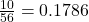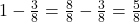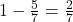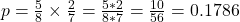## In a chess club the probability that Shaun will beat Mike is 3/8 . The probability that Shaun will beat Tim is 5/7 . (Assume all

Question

In a chess club the probability that Shaun will beat Mike is 3/8 .
The probability that Shaun will beat Tim is 5/7 .
(Assume all the games are independent of one another)
If Shaun plays 1 game with Mike and then 1 game with Tim, what is the probability that Shaun loses both games?

in progress 0
6 months 2021-07-20T02:59:19+00:00 1 Answers 40 views 0probability that Shaun loses both games

Step-by-step explanation:

Games are independent, so we find each separate probability, and multiply them.

In a chess club the probability that Shaun will beat Mike is 3/8.

Soprobability that Shaun loses.

The probability that Shaun will beat Tim is 5/7 .

Soprobability that Shaun loses.

What is the probability that Shaun loses both games?

This is:probability that Shaun loses both games